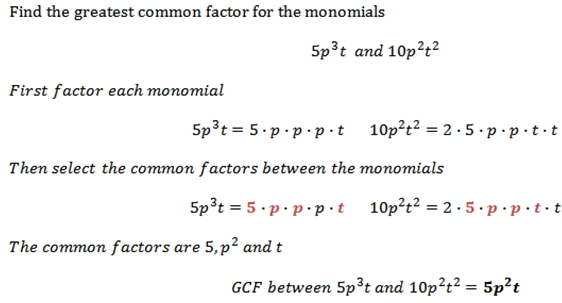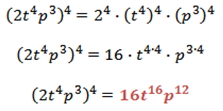Monomials

Monomials and the GCF

A monomial is a number, a variable raised to a whole number, or a product of a number and one or more variables.

Examples: 12x2y,   3mn,   64w3y2

In algebra, a polynomial is the sum of two or more monomials. A polynomial with 2 terms is knows as binomial, and it's the sum of two monomials.

Examples:   (12x2y2 + 3x3y2);   (12m + 64)   and   (3- 64p3q)

A polynomial with 3 terms is known as a trinomial; a polynomial with 4 terms is known as a quadrinomial and so on.

Factoring Monomials

To factor a monomial means to write the monomial as a product of its factors.

Finding the greatest common factor (GCF)Product of Powers Property

To raise a monomial to a power, multiply exponents for each variable by the external power; and raise each numeric factor to the external power.

Example:

Simplify:  (2t 4p3)4